PHYSICS

 Time: ONE Hour Max. Marks: 60

• Answers must be written either in English or the medium of instruction of the candidate in high school.
• Answer all the questions in the booklets provided for the purpose.
• There will be no negative marking.
• The relevant working in arriving at an answer has to be shown wherever required.
• Use of calculators or graph papers is not permitted.
• All questions carry equal marks
1. Two boats A and B can each travel at 4 m/s relative to a stream. At a certain moment they are 1.2 km apart with A going down stream and B upstream. The stream is flowing at 2 m/s. How far apart are they 20 s after they cross each other. If the engine of boat B were to fail at the moment the boats cross and the boat B were to travel with the stream, find the distance between the boats 30 s after the crossing.

2. Two long trains are moving in opposite directions on parallel paths. One at 36 kmph and the other at 72 kmph. A person sitting in the slower train sees the faster train taking 20 s to pass him. Find the length of the faster train. If an observer in the faster train sees the slower train passing him in 10 s find the length of the slower train. How long do the trains take to cross each other.

3. Reaction time of a driver is the time interval between the moment the driver sights an obstacle and the moment he applies the brakes. During this time the car travels with a constant speed at which he is driving and gets nearer to the obstacle.
A driver has a reaction time of 0.5 s and is travelling at 72 kmph on a straight road. What should be the minimum deceleration produced by the brakes of his car if he should avoid hitting an obstacle  50 m away from the car at the moment he sees it.

4. A toy rocket leaves the ground with zero initial velocity and accelerates for 5 s at 2 m/s2. The fuel is finished at this moment and it continues to move up till its velocity becomes zero. Find the maximum height reached by it and the total time for which it is in the air before returning to the ground.

5. A cylindrical vessel of base area 50 cm2 and height 25 cm is filled with one litre of water. A cube of side 5 cm made of a material of relative density 0.8, is lowered in to the vessel. By how much will the level of water rise. What part of the cube can be seen above water? If the height of the vessel were 22 cm, how much water would have overflown?

 A point object is located as shown. Find the point of convergence or divergence of the rays that pass through the lens.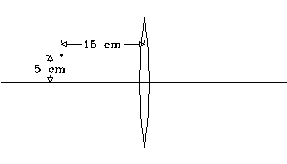Also find the point of convergence or divergence of the rays from the object after they pass through the lens, if the object is moved parallel to the principal axis and away from the lens by 10 cm. Focal length of the lens is 20 cm. Graphs of sine of angle of incidence in medium 1 (on the x –axis) and sine of angle of refraction in medium 2 ( on the y – axis ) as ray of light travels from medium 1 to 2 are as shown.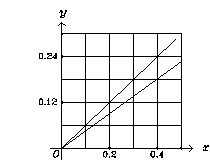One of the graphs is for violet colour and the other is for red. What are the refractive indices for the red and viloet colours of medium 1 with respect to medium 2. One of the graphs is for violet colour and the other is for red. What are the refractive indices for the red and viloet colours of medium 1 with respect to medium 2.
1. Two small spheres have their radii in the ratio 1:2 and are some distance apart. They have charges of -  6 m C and 12 m C and exert a force of 12 N on each other. The spheres are now connected by a conducting wire and the distance between them is halved Assume the total charge on them is shared in the direct ratio of their radii after the connection and find the new force between them.

2. A long conductor is carrying current towards north. A moving charged particle A located at a certain instant vertically above it has a velocity directed southward and the force on it is directed upward. What is the sign of the charge on A. Find the direction of the force on an oppositely charged particle B moving northward and located at a certain instant to the east of the conductor.

 Sections ACB and ADB of the wire shown have thicknesses in the ratio 1:2 and are made of same material. A current of 7 A enters at A. Find the currents in the sections ADB and ACB. If the potential difference between A and B is 18 V, find the resistances of the wires ACB and ADB.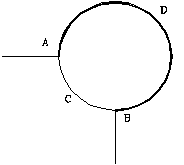1. A satellite is revolving in a circular orbit in the equatorial plane and completes one revolution in 90 min relative to the centre of the earth. What is the time interval between the successive crossings of the satellite over a point on the equator if the satellite is spinning from a) west to east b) east to west.

1. Find the energy in joules per reaction of the following nuclear reaction.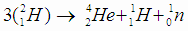the masses of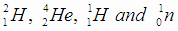are 2.0141 u, 4.0026 u, 1.0078 u and 1.0087 u respectively. A city consumes 1016 J of energy per year. How many kg of sea water is needed per year, if 0.015% of hydrogen atoms in sea water are deuterium.